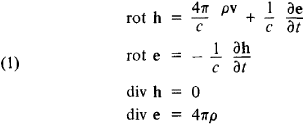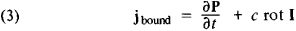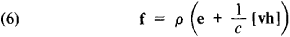# Lorentz-Maxwell Equations

The following article is from The Great Soviet Encyclopedia (1979). It might be outdated or ideologically biased.

## Lorentz-Maxwell Equations

(Lorentz equations), the fundamental equations of classical electrodynamics describing the microscopic electromagnetic fields generated by individual charged particles. The Lorentz-Maxwell equations underlie the electron theory (microscopic electrodynamics) set up by H. A. Lorentz at the end of the 19th century and the beginning of the 20th. Matter (the medium) in this theory is considered as a set of electrically charged particles (electrons and atomic nuclei) moving in a vacuum.

In the Lorentz-Maxwell equations, an electromagnetic field is described by two vectors: the intensities of the microscopic fields —e for the electric field and h for the magnetic field. In the electron theory, all electric currents are purely convective, that is, caused by the motion of charged particles. The current density is j = pv, where p is the charge density and v is the velocity of the charge.

The Lorentz-Maxwell equations were obtained as a result of a generalization of the macroscopic Maxwell equations. In differential form in the absolute Gaussian system of units they arewhere c is the velocity of light in a vacuum.

According to the electron theory, equations (1) accurately describe the fields at any point in space (including interatomic and intraatomic fields and even the fields within an electron) at any point in time. In a vacuum they coincide with the Maxwell equations.

The microscopic field intensities e and h change very rapidly in space and time and are not immediately suited to the description of electromagnetic processes in systems that contain a large number of charged particles (that is, in macroscopic material bodies). But it is precisely these macroscopic properties that are of interest, for example, in electrical and radio technology. For example, for a current of 1 A, about 1019 electrons pass through a cross section of a conductor in 1 sec. It is impossible to follow the motion of all of these particles and to calculate the fields that they generate. Therefore, we resort to statistical methods, which make it possible, on the basis of certain model concepts of the structure of matter, to establish relations between the average values of the electric and magnetic field intensities and the averaged values of the charge and current densities.

The averaging of microscopic quantities is carried out for space and time intervals that are large in comparison with microscopic intervals (of the order of atomic dimensions and the time required for electrons to revolve around the nucleus) but are small in comparison with the intervals over which the macroscopic characteristics of an electromagnetic field change appreciably (for example, in comparison with the length and period of an electromagnetic wave). Such intervals are said to be “physically infinitesimal.”

The averaging of the Lorentz-Maxwell equations leads to the Maxwell equations. Here, it turns out that the average value of the intensity of the microscopic electric field ē is equal to the field intensity in Maxwell’s theory—ē = E—and the value of the intensity of the microscopic magnetic field hē is equal to the vector of magnetic induction—hē = B.

In Lorentz’ theory all charges are divided into free charges and bound charges (which are part of electrically neutral atoms and molecules). It can be shown that the density of the bound charges is given by the polarization vector P (the electric dipole moment of unit volume of the medium):

(2) ρbound = —div P

The current density of the bound charges depends on the magnetization I (the magnetic moment of unit volume of the medium) in addition to the polarization vector:Vectors P and I characterize the electromagnetic state of the medium. By introducing two auxiliary vectors—the vector of electrical induction

(4) D – E + 4πP

and the vector of magnetic field intensity

(5) H = B – 4πI

we obtain Maxwell’s macroscopic equations for the electromagnetic field in matter in conventional form.

In addition to equations (l) for the microscopic fields, an expression for the force acting on charged particles in an electro-magnetic field should be added to the fundamental equations of the electron theory. The volume density of this force (the Lorentz force) isThe averaged value of the Lorentz forces acting on the charged particles that make up a body determines the macroscopic force that acts on the body in an electromagnetic field.

Lorentz’ electron theory has made it possible to clarify the physical meaning of the fundamental constants that enter into the Maxwell equations and that characterize the electrical and magnetic properties of matter. Certain important electrical and optical phenomena, such as the normal Zeeman effect, the dispersion of light, and the properties of metals, were predicted or explained on the basis of this theory.

The laws of the classical electron theory no longer hold for very small space-time intervals. In this case, the laws of the quantum theory of electromagnetic processes—quantum electrodynamics—are valid. The Lorentz-Maxwell equations serve as the basis for the quantum generalization of the theory of electromagnetic processes.

### REFERENCES

Lorentz, H. A. Teoriia elektronov i ee primenenie k iavleniiam sveta i teplovogo izlucheniia, 2nd ed. Moscow, 1953. (Translated from English.)
Becker, R. Elektronnaia teoriia. Leningrad-Moscow, 1936. (Translated from German.)
Landau, L. D., and E. M. Lifshits. Teoriiapolia. Moscow, 1967. (Teoreticheskaia fizika, vol. 2.)

G. IA. MIAKISHEV

The Great Soviet Encyclopedia, 3rd Edition (1970-1979). © 2010 The Gale Group, Inc. All rights reserved.
Site: Follow: Share:
Open / Close